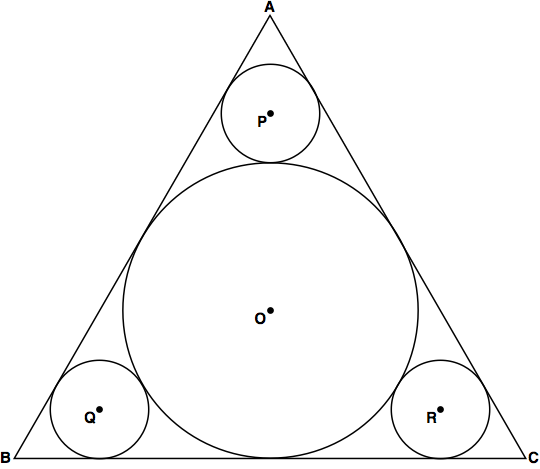# Circles in a Triangle 02

Geometry Level 3In the figure above, three small circles have equal radii of $r=4$ and one big circle centered $O$ has radius $R$. Given an $\triangle ABC$ is equilateral. If the side of the triangle is $a$, then find $\dfrac a{\sqrt3}$.

×